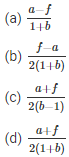# Kinematics MCQ for NEET

NEET  Physics is the scoring paper in the medical entrance examination. Here, you will discover the NEET Physics MCQ Questions for all Concepts as per the latest syllabus. Practice more on a regular basis with these NEET Physics objective questions on air pollution and improve your subject knowledge & problem-solving skills along with time management. NEET Physics Kinematics Multiple Choice Questions make you feel confident in answering the question in the exam & increases your scores to high.

## MCQ on Kinematics

1. Consider two cars M and N. These cars start from the same point and travel in a straight line such that their positions are xM(t) = at + bt2 and xN(t) = ft – t2. Determine the time at which both the cars are having the same velocityAnswer: (b) $$\frac{f-a}{2(1+b)}$$

2. Below is the distance-time curve traced by the particle. What is the maximum instantaneous velocity of the particle around the point(a) D
(b) B
(c) A
(d) C

3. Below is the graph of a body whose initial velocity is v0. The body travels through a straight line with acceleration as shown in the graph. Determine the maximum velocity of the body.(a) v0−4at0
(b) v0+4at0
(c) v0+(at0)
(d) $$v_{0}+\frac{9}{2}\left(a t_{0}\right)$$

Answer: (d) $$v_{0}+\frac{9}{2}\left(a t_{0}\right)$$

4. What will be the shape of the x→y graph, if the relation between time and displacement of the moving body is t = 2αx2 where α is constant.
(a) Circle
(b) Straight line
(c) Parabola
(d) Hyperbola

5. Which of the following statement is true if a body moves in a semicircular track whose radius is R
(a) 2R is the displacement of the body
(b) πR is the distance travelled by the body
(c) Both (a) and (b) are correct
(d) None of the above

Answer: (c) Both (a) and (b) are correct

6. What does the area under acceleration-time graph represent for any given time interval
(a) Final velocity
(b) Distance travelled
(c) Change in the velocity in that time interval
(d) Displacement of the particle

Answer: (c) Change in the velocity in that time interval

7. When can we say that the resultant of the two vectors is maximum
(a) When the vectors are acting in the opposite direction
(b) Both the vectors are acting in the same direction
(c) When the vectors are at right angles
(d) When the vectors are parallel to each other

Answer: (b) Both the vectors are acting in the same direction

8. From the below, select the unit vector along $$\hat{i}+\hat{j}$$Answer: (a) $$\frac{\hat{i}+\hat{j}}{\sqrt{2}}$$
9. A particle with radius R is moving in a circular path with constant speed. The time period of the particle is T. Calculate the time for the following after $$t=\frac{T}{6}$$. What is the average velocity of the particleAnswer: (c) $$\frac{6 R}{T}$$